Relativity and Cosmology Blog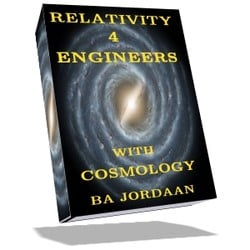# Relativity and Cosmology

This is a Blog on relativity and cosmology for engineers and the like. My website "Relativity-4-Engineers" has more in-depth stuff.

Comments/questions of a general nature should preferably be posted to the FAQ section of this Blog (http://cr4.globalspec.com/blogentry/316/Relativity-Cosmology-FAQ).

A complete index to the Relativity and Cosmology Blog can be viewed here: http://cr4.globalspec.com/blog/browse/22/Relativity-and-Cosmology"

Regards, Jorrie

 Previous in Blog: De Sitter Cosmic Model Revival Next in Blog: Our Observable Universe a Transient?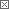# Cosmological Calculator Update

Posted June 18, 2012 10:00 AM by Jorrie

I have often referred to the Cosmo-Calculator in the past. Here is a Blog post dedicated to this ''workhorse''. The picture below is a cropped screenshot, so rather look at the real-thing under the link.

You may wonder: why an update? Is there something new?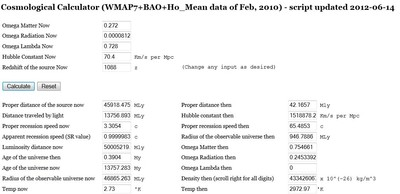Not really, but there are a few extra bits and pieces of information in the updated calculator that may be of interest to some readers. The change was motivated by a comment (on another Blog) that enquired about using the standard Special Relativity (SR) Doppler shift to explain cosmological redshift. My immediate reaction was that it is invalid because SR only applies in flat (Minkowski) spacetime. The cosmos is in all probability 'flat' in term of space, but not in terms of spacetime. The simple reason is that there are gravity and vacuum energy (possibly) operating on the cosmic scales.

Then I remembered that in my eBook Relativity-4-Engineers, I used exactly that: the SR formula for Doppler shift as related to cosmic redshift. In chapter 16, I noted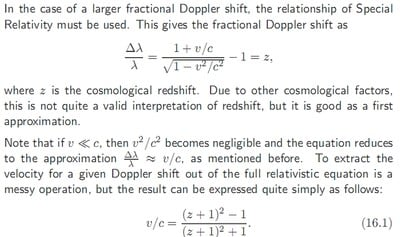The question is: why is this a good approximation of a cosmological recession speed? My best answer is that it is partly due to a coordinate system choice and partly due to the quasi-flatness of the present values of the ΛCDM cosmic model.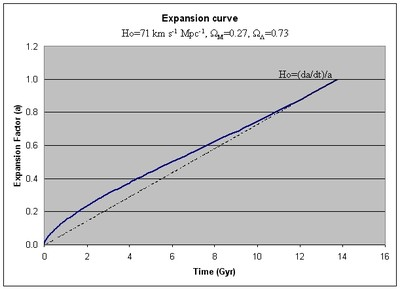The solid curve represents ΛCDM and the dotted line is for a simple linear expansion law, where a = t/THubble, with THubble the inverse of the Hubble constant H0 (in suitable units). From the recession speed v/c, one gets distance D, due to Hubble's Law: v/c = H0D.

H0 is normally expressed in units of km/s per Megaparsec (Mpc), i.e. recession speed per unit distance. Distances in Mpc normally means co-moving or 'proper' cosmological distances, telling us how far the source is from us today, assuming that one could hypothetically stop expansion completely and then see how long light would take to traverse the distance (or measure it with our standard meter sticks).

However, in the SR-Doppler-redshift interpretation, that distance is look-back distance, derived directly from how long it took light to travel the distance in an expanding space. It usually has the units light-year, for obvious reasons. It is simply a different coordinate system than the usual cosmological choice, but there is nothing wrong with it.

In a way, this SR interpretation is easier to comprehend than the usual co-moving interpretation. The SR value is usually qualified with the term apparent (i.e. apparent recession speed/velocity). The latter is just referred to as recession speed or sometimes proper recession speed, due to its connection with proper distance. At the risk of confusion, I will measure both distance types in million light-years (Mly), but will name them appropriately.

Now back to the cosmo-calculator, as pictured above, or under the hotlink provided. The relationship between proper recession speed and apparent (SR) recession speed is immediately visible. For z=1088 (CMB redshift, the farthest we can optically observe), the present proper distance is 45,918 - i.e. the region of early space were the radiation originated, is presently that far from us. Its proper recession speed 'now' is 3.3c. The proper recession speed 'then' was about 65c. The present look-back distance is 13,757 Mly, i.e. less that 0.4 Mly from the theoretical look-back distance of the BB. The apparent recession speed of the region is 0.999998 c.

As I said above, the latter is perhaps the more comprehensible value. The more distant the source (higher z), the closer to c the apparent recession speed becomes, yet it never reaches c. The proper recession speed 'now' levels off at around 3.4c, as we reach the horizon of the observable universe. However, the proper recession speed 'then' increases without limit against z, according to the standard ΛCDM model.

To wrap up the output, I have added the average temperatures of the CMB 'now' and 'then', which are simply related by a factor 1/a = (1+z). You can check by inputting a redshift of 9 and note that the temperature 'then' is 10 times larger than 'now'.

Any feedback would be appreciated.

-J

 You can read chapter 16 here; you will find the complete Relativity-4-Engineers here.

 One Mpc is about 3.26 light-year. See Wiki for more definition on cosmic distances.

A successful quantum gravity theory may avoid these infinities - and it may imply a 'bounce' rather than a 'bang'.

Interested in this topic? By joining CR4 you can "subscribe" to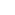#1

###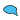Re: Cosmological Calculator Update

06/18/2012 11:27 PM

Jorrie-

I keep coming across this factor 1/sqrt(1-v²/c²)- first encountered as the Lorentz contraction, and apparently the same factor is used to define time dilation. I see it popping up in your formulas today. It is obvious from the equation that achieving the speed of light is impossible. I am aware of the mathematical derivation of the Lorentz contraction, but I have never found a physical explanation of why this particular relationship is valid. I am not questioning the validity. I am just curious as to WHY it holds...Any ideas?#2

###Re: Cosmological Calculator Update

06/19/2012 1:01 AM

The factor 1/sqrt(1-v²/c²) is known as the 'gamma factor', which represents both relativistic time dilation and Lorentz contraction, as you said.

I prefer to think of it as time dilation, because it can be directly measured as such, e.g. particle half-times in accelerators, or clocks in orbit. AFAIK, Lorentz contraction has not been directly measured, but it is implied by time dilation.

What is the mechanism behind time dilation? It is the inter-relationship of space and time, which (over-) simply stated means: when a perfect clock is forced to move in space, it 'moves' less in time. It is measurable by moving a clock in a spatially closed path (circle, square, whatever) and note that its elapsed time is less that the elapsed time on an identical clock that has remained at rest. But, if you calculate the spacetime interval: S2 = Δr2 - cΔt2 for each clock, you will find them to be identical.

So, more precisely stated, the mechanism is the invariance of the spacetime interval.

-J

__________________
"Perplexity is the beginning of knowledge." -- Kahlil Gibran
Guru

Join Date: Dec 2006
Location: North America, Earth
Posts: 4399#3

###Re: Cosmological Calculator Update

06/19/2012 7:31 PM

Jorrie,

Am I correct in assuming this uses the ΛCDM model? Have you thought about doing one for another model (such as de Sitter) to see how they compare?

-S

__________________
“I would rather have questions that can't be answered than answers that can't be questioned.” - Richard Feynman#4

###Re: Cosmological Calculator Update

06/19/2012 11:45 PM

S,

Yes, the calculator is designed for the ΛCDM model, but since the de Sitter model is just one with zero and radiation and matter, it will calculate it with matter and radiation density set to zero. It will also calculate the Einstein-de Sitter case, with zero radiation or vacuum energy and for that matter the Milne case, with all energies set to zero.

-J

__________________
"Perplexity is the beginning of knowledge." -- Kahlil Gibran